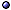In General > s.a. chaos; MaxEnt (Maximum Entropy Principle); history of physics; statistical mechanics; thermodynamics.
* Thermodynamic entropy: An extensive thermodynamical function of state which is non-decreasing for adiabatic transformations (constant in reversible ones); In most systems, it grows monotonically with E; The concept was introduced by Clausius in the 1860s and the Clausius entropy is defined, up to an additive constant, by the relation dS = dQ/T.
* Statistical interpretation: A measure of how spread out a classical distribution function or quantum density ρ matrix is; For a system that can be in Ω equiprobable states the Boltzmann entropy is SB = kB ln Ω; More generally, for a classical probability distribution the Gibbs entropy is SG = −kB i pi ln pi, and for a quantum density matrix the von Neumann entropy is S = −kB tr ρ ln ρ; > s.a. types of entropies.
* And classical / quantum mechanics: In classical mechanics one assumes entropies are finite, but if one takes the $$\hbar$$ → 0 limit of any quantum entropy expression, this diverges; So classical thermodynamics is not the classical limit of quantum statistical mechanics, it knows about quantum mechanics.
@ Articles, II: Han et al cm/97, AJP(99)jan [tracing over degrees of freedom]; Styer AJP(00)dec [examples and meaning]; Lieb & Yngvason PT(00)apr [without thermodynamics]; Styer AJP(08)oct [and evolution]; Salagaram & Chetty AJP(11)nov [computation].
@ Books: Martin & England 81; Denbigh & Denbigh 85; Leff & Rex ed-90; Dugdale 96 [II]; Scully & Scully 07 [Maxwell's demon, information, and the quantum]; Grandy 08 [and probability]; Ben-Naim 16 [I].
@ General references: Wehrl RMP(78); in Sorkin PRL(86); Mackey RMP(89) [increasing entropy and ergodicity]; Lavenda et al NCB(95); Leff AJP(96)oct [and energy sharing]; Levy math/06 [mathematical interpretation]; Kozlov & Treshchev TMP(07) [coarse-grained]; Bernstein a1109 [pedagogical]; Baumgartner FP(14)-a1206 [axiomatic characterization and derived facts]; Zanchini & Beretta Ent(14)-a1403 [general definition]; Phillips TPT(16) [entropy as the spread of energy]; Jakšić a1806 [and information theory]; Kycia a1908 [formal overview].
@ Conceptual: Leff FP(07) [language and interpretation]; Topsoe a0807 [entropy and truth vs belief]; Timberlake TPT(10) [statistical interpretation]; Swendsen AJP(11)apr [definitions and meaning]; Baez et al Ent(11)-a1106 [characterization in terms of information loss]; Davey PhSc(11) [and probability]; Swendsen FP(12) [argument for probabilistic definition]; Dieks a1209-in [thermodynamical vs statistical-mechanical definitions]; Benguigui EJP(13)-a1209 [different definitions]; Caticha M&M-a1412 [entropic inference as a general framework for reasoning under conditions of uncertainty]; in Oltean et al PRD(16)-a1607 [nature of S]; Toffoli Ent(16)-a1705 [and "honest entropy"]; Styer TPT(19)sep [entropy isn't always disorder]; Weaver a2004 [Boltzmann and Clausius entropy]; Brandsen et al a2103 [perspective from games of chance].
@ And observation: Steane NJP(16)-a1510 [determining absolute entropy without quantum theory or the third law].

Related Concepts and Generalizations > s.a. Coarse Graining; Disentropy; dissipation; Entropic Dynamics; Homogeneity.
@ And area law: Mäkelä gq/06-GRF; Masanes PRA(09)-a0907 [for low-energy states].
@ Other properties: Kay & Kay JPA(01)mp/98 [strong subadditivity and volume]; Touchette AJP(08)jan [(non)-concavity].
@ Subsystems: Hotta & Joichi PLA(99)cm [additivity]; Baumgartner JPA(02)mp/01; Dupuis et al a1607 [additivity, "accumulation"].
@ Entropy current: Bhattacharyya JHEP(14)-a1403, JHEP(14) [and the equilibrium partition function]; Banerjee et al JHEP(14) [non-relativistic fluid].
@ Beyond equilibrium situations: Beretta & Zanchini in(11)-a1010; Beretta & Zanchini a1411, AAPP(19)-a1911 [and few-particle systems]; > s.a. non-equilibrium thermodynamics.
@ And time: Hotke-Page & Page in(92) [clock time]; Ben-Naim 16 [no relationship].
@ Entropy production and arrow of time: Parrondo et al NJP(09); Martyushev & Shaiapin Ent(16)-a1605; Mintchev & Sorba a2003-proc.
@ Related topics: Hall PRA(99)qp/98 [geometric, ensemble volume]; Leff AJP(99)dec [dimensionless]; Dover PhyA(04) [and scaling laws]; Hanel et al PNAS(12)-a1211 [generalized, for systems violating the Shannon-Khinchin axioms]; Lieb & Yngvason PRS(14)-a1403 [entropy meters and the entropy of non-extensive systems]; Plastino et al PhyA(14) [partition function and entropy from thermodynamics]; Chafaï AFST(15)-a1405 [aspects of entropy in different disciplines].Related topics: see entropy bounds; entropy in quantum theory; types of entropies and entanglement entropy.

Systems
> Gravity-related: see black-hole entropy; gravitational thermodynamics; horizons.
> Other systems: see casimir effect [negative entropies]; cell complex; cellular automaton; networks; thermodynamic systems; tilings.